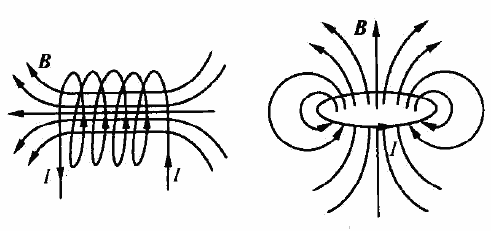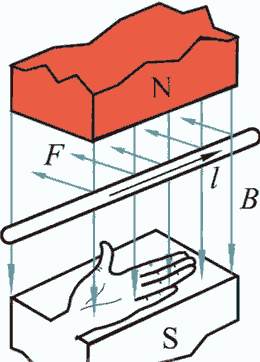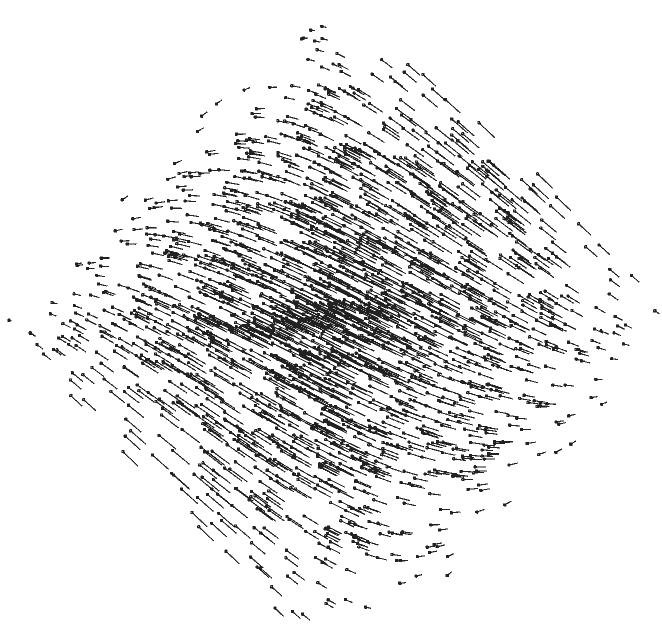# 四维世界（七）：电磁学

/** 这篇文章我打算专注一下四维世界（四维空间+一维时间=五维时空）里的物理学——电磁学。这些都是假设这个世界也符合我们世界中的一些物理规律的理论推导。虽然它电磁学不像牛顿力学那么容易让人接受，但这个类比也是无瑕兹而且很自然的（你也可以认为一切都是扯淡）。我们不讨论量子力学等其他物理，因为它们在四维空间中的推广有严重问题。 **/

## 静电场$$F={kQ_1Q_2\over r^2}$$$$F={kQ_1Q_2\over r^3}$$

/**注意我们默认牛顿三定律都成立，也就是说力的单位还是$\mathrm{kg/(m/s^2)}$，这个次数不会变成三次。那是否存在力的单位是$\mathrm{kg/(m/s^3)}$或$\mathrm{kg/(m/s)}$的世界呢？我也思考过，那些世界非常疯狂！但是跟四维无关所以以后单独讨论。**/

## 电流## 恒定磁场## 从安培力与洛伦兹力入手$$\boldsymbol{F}=I\boldsymbol{l}\times \boldsymbol{B}$$## 磁偶极子与指南针

### 环形电流的力矩

• 当磁场平行于线圈时，线圈上任何一个点都不受力
• 当磁场绝对垂直于线圈时，线圈上每点受力需要同时垂直于线圈导线与磁场，所以只能是沿线圈半径方向，导致线圈有扩大或收缩的趋势，但不会使线圈转动。
• 当磁场半平行半垂直于线圈时（即三维空间那种二面角90°）结论是线圈会受到合力矩，有向顺着磁场的方向转动的趋势。1. 我们来算算力矩：选取线圈中心为作用点，B点的力臂沿y方向，B点受力沿w方向，三维的力矩垂直于两者，推广到四维力矩也该垂直，所以是xz方向。而力矩的方向是转轴的方向，垂直于旋转平面，所以这个力矩的作用效果是让线圈在yw方向上转动。
细心的话你会发现一个问题：既然B点的力臂沿y方向，B点受力沿w方向，效果为让线圈在yw方向上转动，为什么我们的力矩还非得规定是与它们垂直的xz方向？直接规定成yw方向不更好吗？确实，这个滥用垂直方向倒来倒去的问题我们在说二维世界的磁场时也遇到过。三维空间由于可以用更简单的法向量表示平面，所以才会用莫名其妙的垂直于旋转平面方向作为力矩方向。这也暗示了三维空间的磁场可能定义为跟磁场向量垂直且大小相同的2-向量更加合适，不仅省去繁琐的左右手定则，也统一了不同维度下磁场的形式，比如二维磁场也不是标量，而是2-向量，这个2-向量只能平行于二维空间所在的平面，所以只有一个分量，感觉上就是标量。但为了保持跟中学物理知识的一致性，我们还是用那种正常的定义（其实是三维人偏见的定义），用$\boldsymbol{B}^*$表示这种绝对垂直版的更好的定义。
2. 直接把力在xyw空间画出来用眼睛看：我们除了知道旋转发生在yw平面上（或说绕xz轴平面旋转），还能看到线圈有往yw面旋转的趋势。而之前分析过yw面与磁场绝对垂直，确实是一个平衡位置。

### 地磁场与指南针## 四维版麦克斯韦方程？

### 安培环路定理

$$\oint_l\boldsymbol{B}\cdot \boldsymbol{dl}=\mu_0 (\boldsymbol{I}+\iint_D \epsilon_0\frac{\partial \boldsymbol{E}}{\partial t}\cdot \boldsymbol{dn})$$

$$\boldsymbol{B}(A)-\boldsymbol{B}(B)=\mu_0 (\boldsymbol{I}+\int_D \epsilon_0\frac{\partial \boldsymbol{E}}{\partial t}\cdot \boldsymbol{dn})$$$$\oint_S\boldsymbol{B}\cdot \boldsymbol{dS}=\mu_0 (\boldsymbol{I}+\iiint_D \epsilon_0\frac{\partial \boldsymbol{E}}{\partial t}\cdot \boldsymbol{dn})$$

### 法拉第电磁感应定理

$$\oint_l\boldsymbol{E}\cdot \boldsymbol{dl}=-\iint_D \frac{\partial \boldsymbol{B}}{\partial t}\cdot \boldsymbol{dn}$$

$$\oint_l\boldsymbol{E}\cdot \boldsymbol{dl}=-\iint_D \frac{\partial B}{\partial t}\cdot dxdy$$$$\oint_l\boldsymbol{E}\cdot \boldsymbol{dl}=-\iint_D \frac{\partial \boldsymbol{B}^*}{\partial t}\cdot \boldsymbol{dS}$$

### 高斯定理

$$\oint_S\boldsymbol{B}\cdot\boldsymbol{dn}=0$$

$$\oint_S\boldsymbol{B}\cdot\boldsymbol{dS}^*=0$$

$$\oint_S\boldsymbol{B}^*\cdot\boldsymbol{dS}=0$$

$$\epsilon_0\oint_S\boldsymbol{E}\cdot\boldsymbol{dn}=q$$

$$\epsilon_0\oint_V\boldsymbol{E}\cdot\boldsymbol{dn}=q$$

### 四维电磁波

$$\oint_{\partial} \omega=\int_{i}\boldsymbol{d}\wedge \omega$$

• 下面先来写电磁场的高斯定理微分形式：
• $\boldsymbol{d}\boldsymbol{B}^*=(\boldsymbol{d}\boldsymbol{B}^*)^*=0$，磁场$B$的散度为0，为无源场。
• $\epsilon_0(\boldsymbol{d}\boldsymbol{E}^*)^*=\rho$，电场散度为电荷密度。
• 然后是变化的电磁场相互激发：
• $(\boldsymbol{d}\wedge\boldsymbol{E})^*=-\frac{\partial \boldsymbol{B}}{\partial t}$
• $(\boldsymbol{d}\wedge\boldsymbol{B})^*=\mu_0(\boldsymbol{j}+\epsilon_0\frac{\partial \boldsymbol{E}}{\partial t})$

$$(\boldsymbol{d}\wedge(\boldsymbol{d}\wedge\boldsymbol{E})^*)^*=-\mu_0\epsilon_0\frac{\partial^2 \boldsymbol{E}}{\partial t^2}$$

$$(\boldsymbol{d}\wedge(\boldsymbol{d}\wedge \boldsymbol{E})^*)^*= -\boldsymbol{\Delta E}+\text{grad}(\text{div}(\boldsymbol{E}))$$，简直跟三维情况一模一样。由于没有电荷$\rho$，所以右边那项为0，我们终于得到了标准的波动方程：
$$\boldsymbol{\Delta E}-\mu_0\epsilon_0\frac{\partial^2 \boldsymbol{E}}{\partial t^2}=0$$

$$\boldsymbol{\Delta B}-\mu_0\epsilon_0\frac{\partial^2 \boldsymbol{B}}{\partial t^2}=0$$

## 四维波动光学

• 假设电场只有$x$分量，此时电场旋度为零，没有磁场能够激发起这种电场。
• 假设电场只有$y$分量，此时电场旋度漩涡方向为xy方向，如果有zw方向的磁场按正弦波变化就可能激起这种电场，且也按相同频率相位变化。
• 假设磁场只有$xy$分量，此时磁场旋度为零，没有电场能够激发起这种电场。
• 假设磁场只有$yz$分量，此时磁场旋度为w，如果有w方向上的电场按正弦波变化就能够激发起这种电场，且也按相同频率相位变化。